NEET  >  Test: Electromagnetic Induction - 2

# Test: Electromagnetic Induction - 2

Test Description

## 30 Questions MCQ Test Physics Class 12 | Test: Electromagnetic Induction - 2

Test: Electromagnetic Induction - 2 for NEET 2023 is part of Physics Class 12 preparation. The Test: Electromagnetic Induction - 2 questions and answers have been prepared according to the NEET exam syllabus.The Test: Electromagnetic Induction - 2 MCQs are made for NEET 2023 Exam. Find important definitions, questions, notes, meanings, examples, exercises, MCQs and online tests for Test: Electromagnetic Induction - 2 below.
Solutions of Test: Electromagnetic Induction - 2 questions in English are available as part of our Physics Class 12 for NEET & Test: Electromagnetic Induction - 2 solutions in Hindi for Physics Class 12 course. Download more important topics, notes, lectures and mock test series for NEET Exam by signing up for free. Attempt Test: Electromagnetic Induction - 2 | 30 questions in 60 minutes | Mock test for NEET preparation | Free important questions MCQ to study Physics Class 12 for NEET Exam | Download free PDF with solutions
 1 Crore+ students have signed up on EduRev. Have you?
Test: Electromagnetic Induction - 2 - Question 1

### A flux of 1m Wb passes through a strip having an area A = 0.02 m2. The plane of the strip is at an angle of 60º to the direction of a uniform field B. The value of B is

Detailed Solution for Test: Electromagnetic Induction - 2 - Question 1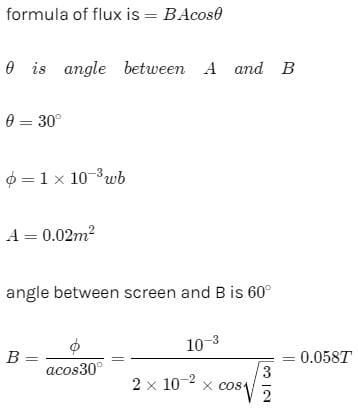Test: Electromagnetic Induction - 2 - Question 2

### The number of turns in a long solenoid is 500. The area of cross-section of solenoid is 2 × 10-3 m2. If the value of magnetic induction, on passing a current of 2 amp, through it is 5 × 10-3 tesla, the magnitude of magnetic flux connected with it in webers will be

Detailed Solution for Test: Electromagnetic Induction - 2 - Question 2

Φ=BAN
=5x10-3x2x10-3x500
=5x10-3x1000x10-3
=5x10-3

Test: Electromagnetic Induction - 2 - Question 3

### A conducting loop of radius R is present in a uniform magnetic field B perpendicular the plane of the ring. If radius R varies as a function of time `t', as R = R0 + t. The e.m.f induced in the loop is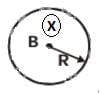Detailed Solution for Test: Electromagnetic Induction - 2 - Question 3

ε=− dϕ​/dt
=−β(dA/dt)

​⇒∣ε∣=βπd(R0​+t)2
=2πβ(R0​+t)
Since inward flux is increasing the EMF will be induced counter clockwise.

Test: Electromagnetic Induction - 2 - Question 4

The instantaneous flux associated with a closed circuit of 10Ω resistance is indicated by the following reaction f = 6t2 _ 5t + 1, then the value in amperes of the induced current at t = 0.25 sec will be

Detailed Solution for Test: Electromagnetic Induction - 2 - Question 4

ϕ=6t2−5t+1
e= dϕ​/dt =12t−5
at t=0.25sec
∣e∣=(12/4−5)=∣3−5∣=2
i=e/R=2/10=0.2A

Test: Electromagnetic Induction - 2 - Question 5

When a magnet is being moved towards a coil, the induced emf does not depend upon

Detailed Solution for Test: Electromagnetic Induction - 2 - Question 5

The magnitude of induced emf is independent of the resistance of the coil.
∴e=−dϕ/dt=−N (d/dt) [(BA)/dt]
Thus, e depends upon N, B and A.
But induced current depends upon R.

Test: Electromagnetic Induction - 2 - Question 6

An electron is moving in a circular orbit of radius R with an angular acceleration a. At the centre of the orbit is kept a conducting loop of radius r, (r < < R). The e.m.f induced in the smaller loop due to the motion of the electron is

Test: Electromagnetic Induction - 2 - Question 7

A field of 5 x 104/p ampere-turns/metre acts at right angles to a coil of 50 turns of area 10-2 m2. The coil is removed from the field in 0.1 second. Then the induced emf in the coil is

Detailed Solution for Test: Electromagnetic Induction - 2 - Question 7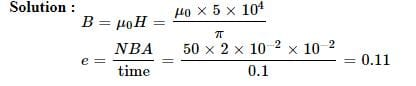Test: Electromagnetic Induction - 2 - Question 8

square wire loop of 10.0 cm side lies at right angles to a uniform magnetic field of 20T. A 10 V light bulb is in a series with the loop as shown in the fig. The magnetic field is decreasing steadily to zero over a time interval Dt. The bulb will shine with full brightness if Dt is equal to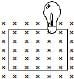Detailed Solution for Test: Electromagnetic Induction - 2 - Question 8

e=−Δϕ/Δt=ϕi−ϕf/Δt
δt=(ϕi−ϕf)/e=(BA−0)/e=(20×10−2)/10
=20×10−3sec=20ms

Test: Electromagnetic Induction - 2 - Question 9

The rate of change of magnetic flux denisty through a circular coil of area 10-2 m2 and number of turns 100 is 103 Wb/m2/s. The value of induced e.m.f. will be

Detailed Solution for Test: Electromagnetic Induction - 2 - Question 9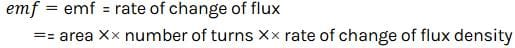Test: Electromagnetic Induction - 2 - Question 10

A long solenoid contains 1000 turns/cm and an alternating current of peak value 1A is flowing in it. A search coil of area of cross-section 1 × 10-4 m2 and having 50 turns is placed inside the solenoid with its plane perpendicular to the axis of the solenoid. A peak voltage of 2p2 × 10-2V is produced in the search coil. The frequency of current in the solenoid will be

Test: Electromagnetic Induction - 2 - Question 11

In an iron cored coil the iron core is removed so that the coil becomes an air cored coil. The inductance of the coil will

Detailed Solution for Test: Electromagnetic Induction - 2 - Question 11

In an iron cored coil the iron core is removed so that the coil becomes an air cored coil. The inductance of the coil will decrease.

Test: Electromagnetic Induction - 2 - Question 12

The magnetic flux through a stationary loop with resistance R varies during interval of time T as f = at (T _ t). The heat generated during this time neglecting the inductance of loop will be

Detailed Solution for Test: Electromagnetic Induction - 2 - Question 12

E=dx/dt =d(a(Tt-t2))/dt=a(T-2t)
I=E/R=a(T-2t)/R
Heat liberated,
△k=I2Rdt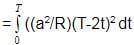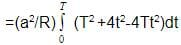=(a2 /R)[T2t+(4t3/3)-2Tt2]T0
=△H=a2T3/3R

Test: Electromagnetic Induction - 2 - Question 13

The dimensions of permeability of free space can be given by

Detailed Solution for Test: Electromagnetic Induction - 2 - Question 13

In SI units, permeability is measured in Henries per meter H/m or Hm−1.
Henry has the dimensions of [ML2T−2A−2].
Dimensions for magnetic permeability will be [ML2T−2A−2]/[L]=[MLT−2A−2]

Test: Electromagnetic Induction - 2 - Question 14

A closed planar wire loop of area A and arbitrary shape is placed in a uniform magnetic field of magnitude B, with its plane perpendicular to magnetic field. The resistance of the wire loop is R. The loop is now turned upside down by 180° so that its plane again becomes perpendicular to the magnetic field. The total charge that must have flowen through the wire ring in the process is

Detailed Solution for Test: Electromagnetic Induction - 2 - Question 14

Induced emf= d(flux)/dt​=R[dq​/dt]
⇒Δq= Δ(flux)​/R=2BA/R​

Test: Electromagnetic Induction - 2 - Question 15

A square coil ABCD is placed in x-y plane with its centre at origin. A long straight wire, passing through origin, carries a current in negative z-direction. Current in this wire increases with time.The induced current in the coil is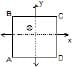Detailed Solution for Test: Electromagnetic Induction - 2 - Question 15

As magnetic field lines due to current carrying wire is along the surface and hence,
ϕ=B.A=BAcos90O=0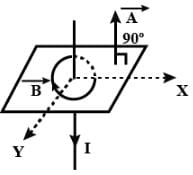Test: Electromagnetic Induction - 2 - Question 16

Two identical coaxial circular loops carry a current i each circulating in the same direction. If the loops approach each other

Detailed Solution for Test: Electromagnetic Induction - 2 - Question 16

As the two identical coaxial circular loops carry a current i each circulating in the same direction, their flux add each other. When the two are brought closer, the total flux linkage increases. By Lenz's law a current will be induced in both the loops such that it opposes this change in flux. As the flux increases, the current in each loop will decrease so as to decrease the increasing flux.

Test: Electromagnetic Induction - 2 - Question 17

In the arrangement shown in given figure current from A to B is increasing in magnitude. Induced current in the loop will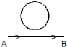Detailed Solution for Test: Electromagnetic Induction - 2 - Question 17

The direction of the induced current is as shown in the figure, according to Lenz’s law which states that the indeed current flows always in such a direction as to oppose the change which is giving rise to it.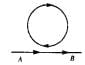Test: Electromagnetic Induction - 2 - Question 18

The north pole of a magnet is brought near a coil. The induced current in the coil as seen by an observer on the side of magnet will be

Detailed Solution for Test: Electromagnetic Induction - 2 - Question 18

The direction of the current will be anticlockwise.

According to Lenz's law, the current in the coil will be induced in the direction that'll oppose the external magnetic field .
As the flux due to the external magnet is increasing ( as the N-pole is brought close ) , the coil will have to induce current in the anticlockwise direction to oppose this increase in flux and not in clockwise direction as that'll end up supporting the external flux.

Test: Electromagnetic Induction - 2 - Question 19

A metal sheet is placed in a variable magnetic field which is increasing from zero to maximum. Induced current flows in the directions as shown in figure. The direction of magnetic field will be -Detailed Solution for Test: Electromagnetic Induction - 2 - Question 19

The direction of these circular magnetic lines is dependent upon the direction of current. The density of the induced magnetic field is directly proportional to the magnitude of the current. Direction of the circular magnetic field lines can be given by Maxwell's right hand grip rule or Right handed cork screw rule.

Test: Electromagnetic Induction - 2 - Question 20

A small conducting rod of length l, moves with a uniform velocity v in a uniform magnetic field B as shown in fig-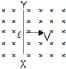Detailed Solution for Test: Electromagnetic Induction - 2 - Question 20

The rod is moving towards the right in a field directed into the page.
Now, if we apply Fleming's right hand rule, then the direction of induced current will be from end X to end Y.
But, according to Lenz's law the emf induced in the rod will be such that it opposes the motion of the rod.
Hence, the actual emf induced will be from end Y to end X. So, the current will also flow from end Y to end X.
Now, using the convention of current end Y should be positive and end X should be negative.
So, correct answer is option b

Test: Electromagnetic Induction - 2 - Question 21

The magnetic field in a region is given by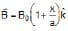. A square loop of edge-length d is placed with its edge along x & y axis. The loop is moved with constant velocity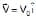. The emf induced in the loop is

Detailed Solution for Test: Electromagnetic Induction - 2 - Question 21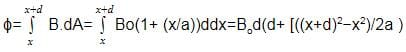ϵ=− dϕ/dt ​=dϕdx​/dx​dt
=Bo​d (2d​/2a)vo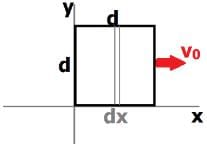Test: Electromagnetic Induction - 2 - Question 22

Consider the situation shown in fig. The resistanceless wire AB is slid on the fixed rails with a constant velocity. If the wire AB is replaced by a resistanceless semicircular wire, the magnitude of the induced current will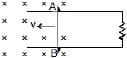Detailed Solution for Test: Electromagnetic Induction - 2 - Question 22

Rate of change of area with remain same even if wire is replaced by semi-circular wire,
∴ induced current will be same as earlier one as I = (e/R) & e i.e. induced emf is function of area because
e = – N (dɸ/dt) = – N (d/dt) (ABcoswt).

Test: Electromagnetic Induction - 2 - Question 23

A conducting square loop of side I and resistance R moves in its plane with a uniform velocity v perpendicular to one of its sides. A uniform and constant magnetic field B exists along the perpendicular to the plane of the loop in fig. The current induced in the loop is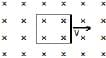Detailed Solution for Test: Electromagnetic Induction - 2 - Question 23

Voltage is induced due to change in the magnetic flux through a closed loop.
Here
Magnetic Flux = ϕ=BA=const.
Hence
Induced EMF = dtdϕ​=0
Induced current =i=E/R=0

Test: Electromagnetic Induction - 2 - Question 24

Two identical conductors P and Q are placed on two frictionless fixed conducting rails R and S in a uniform magnetic field directed into the plane. If P is moved in the direction shown in figure with a constant speed, then rod Q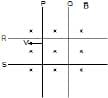Detailed Solution for Test: Electromagnetic Induction - 2 - Question 24

As the conductor P moves away from Q, the area of the loop enclosed by the conductors and the rails increases. This in turn increases the flux through the loop.
EMF will be induced in such a way that the change in flux will be resisted. The induced current will cause Q to move towards P thereby reducing the area and thus the flux back.

Test: Electromagnetic Induction - 2 - Question 25

Two infinitely long conducting parallel rails are connected through a capacitor C as shown in the figure. A conductor of length l is moved with constant speed v0. Which of the following graph truly depicts the variation of current through the conductor with time ?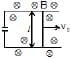Detailed Solution for Test: Electromagnetic Induction - 2 - Question 25

ε=− dϕ​/dt
=−Bl (dx/dt) ​=−Blv0​
This emf should induce the movement of charges creating a current. But due to the attached capacitor, all charges are conserved.
Thus I= dq/dt ​=0
The correct option is C.

Test: Electromagnetic Induction - 2 - Question 26

A thin wire of length 2m is perpendicular to the xy plane. It is moved with velocity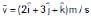through a region of magnetic induction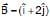Wb/m2. Then potential difference induced between the ends of the wire

Detailed Solution for Test: Electromagnetic Induction - 2 - Question 26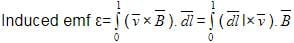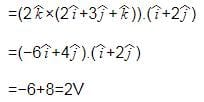Test: Electromagnetic Induction - 2 - Question 27

A long metal bar of 30 cm length is aligned along a north south line and moves eastward at a speed of 10 ms-1. A uniform magnetic field of 4.0 T points vertically downwards. If the south end of the bar has a potential of 0V, the induced potential at the north end of the bar is

Detailed Solution for Test: Electromagnetic Induction - 2 - Question 27

Induced emf =Blv=12V. It is induced in the northward direction by right hand rule (emf=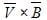)
therefore if the south end of the pole has potential of 0V, the north end will have a potential of 12V.

Test: Electromagnetic Induction - 2 - Question 28

A conducting rod moves with constant velocity v perpendicular to the long, straight wire carrying a current I as shown compute that the emf generated between the ends of the rod.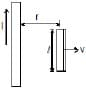Detailed Solution for Test: Electromagnetic Induction - 2 - Question 28

The magnetic field near the conducting rod due to long current carrying wire is
B= μ0​I/2πr ​ (using Ampere's law, ∫B.dl=μ0​I)
Now, induced emf due to moving rod is e=Blv= (μ0​I/2πr) ​×lv= μ0​vIl/2πr

Test: Electromagnetic Induction - 2 - Question 29

A square loop of side a and resistance R is moved in the region of uniform magnetic field B (loop remaining completely insidefield), with a velocity v through a distance x. The work done is

Detailed Solution for Test: Electromagnetic Induction - 2 - Question 29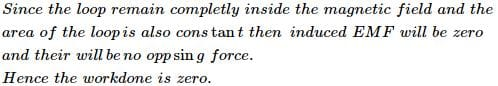Test: Electromagnetic Induction - 2 - Question 30

A rod closing the circuit shown in figure moves along a U shaped wire at a constant speed v under the action of the force F. The circuit is in a uniform magnetic field perpendicular to the plane. Calculate F if the rate of heat generation in the circuit is Q.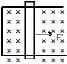## Physics Class 12

157 videos|452 docs|213 tests
 Use Code STAYHOME200 and get INR 200 additional OFF Use Coupon Code
Information about Test: Electromagnetic Induction - 2 Page
In this test you can find the Exam questions for Test: Electromagnetic Induction - 2 solved & explained in the simplest way possible. Besides giving Questions and answers for Test: Electromagnetic Induction - 2, EduRev gives you an ample number of Online tests for practice

## Physics Class 12

157 videos|452 docs|213 tests

### How to Prepare for NEET

Read our guide to prepare for NEET which is created by Toppers & the best Teachers